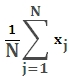# SQL Server average (arithmetic mean) function

Updated: 6 August 2010

Use AVERAGE to return the average (arithmetic mean) for a dataset. The equation for AVERAGE is:Syntax
SELECT [wctStatistics].[wct].[AVERAGE] (
<@Values_TableName, nvarchar(4000),>
,<@Values_ColumnName, nvarchar(4000),>
,<@Values_GroupedColumnName, nvarchar(4000),>
,<@Values_GroupedColumnValue, sql_variant,>)
Arguments
@Values_TableName
the name, as text, of the table or view that contains the values to be used in the AVERAGE calculation.
@Values_ColumnName
the name, as text, of the column in the table or view specified by @Values_TableName that contains the known x values to be used in the AVERAGE calculation.
@Values_GroupedColumnName
the name, as text, of the column in the table or view specified by @Values_TableName which will be used for grouping the results.
@Values_GroupedColumnValue
the column value to do the grouping on.

Return Types
float
Remarks
·         AVERAGE is the arithmetic mean and is calculated by adding a group of numbers and then dividing by the count of those numbers. For purposes of this calculation, NULL values are not included.
·         No GROUP BY is required with this function even though it produces aggregated results.
·         AVERAGE may be used on normalized and de-normalized table, but for more complex queries, consider user AVERAGE_q
Examples

Using the normalized table #s1, calculate the average student grade for the Math.

This produces the following result

----------------------
87.822

(1 row(s) affected)

Using the normalized table #s1, calculate the average grade for each subject

from #s1 s

This produces the following result

subject
-------------------------------------------------- ----------------------
Foreign Language                                   84.897
History                                            91.72
Literature                                         91.72
Math                                               87.822
Science                                            89.77

Using the normalized table #s1, calculate the average grade for each student

from #s1 s

This produces the following result

student
-------------------------------------------------- ----------------------
Student 01                                         97.506
Student 02                                         94.734
Student 03                                         93.59
Student 04                                         92.776
Student 05                                         92.114
Student 06                                         91.542
Student 07                                         91.028
Student 08                                         90.554
Student 09                                         90.104
Student 10                                         89.672
Student 11                                         89.25
Student 12                                         88.83
Student 13                                         88.414
Student 14                                         87.986
Student 15                                         87.55
Student 16                                         87.092
Student 17                                         86.604
Student 18                                         86.076
Student 19                                         85.478
Student 20                                         84.776
Student 21                                         83.882
Student 22                                         82.526

(22 row(s) affected)

### SupportCopyright 2008-2023 Westclintech LLC         Privacy Policy        Terms of Service